### Choose language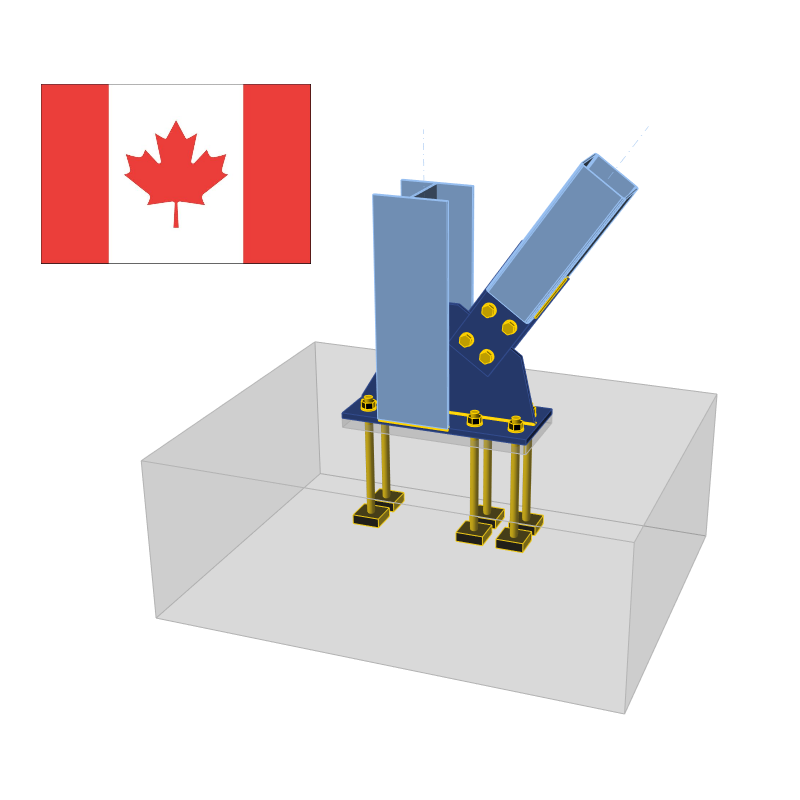# Check of components according to CISC (Canadian standards)

$$CBFEM method combines the advantages of the general finite element method and the standard component method. The stresses and internal forces calculated on the accurate CBFEM model are used in checks of all components.Components are designed according to the Canadian standard (Canadian Institute of Steel Construction, CISC) S16-14 Design of steel structures and CSA A23.3 Design of concrete structures. Design check of plates according to CISC The resulting equivalent stress (HMH, von Mises) and plastic strain are calculated on plates. When the yield strength (multiplied by resistance factor for structural steel ϕ = 0.9, which is editable in Code setup) on the bilinear material diagram is reached, the check of the equivalent plastic strain is performed. The limit value of 5 % is suggested in Eurocode (EN1993-1-5 App. C, Par. C8, Note 1), this value can be modified in Code setup but verifications were done for the recommended value.Plate element is divided into 5 layers and elastic/plastic behavior is investigated in each of them. The program shows the worst result from all of them. The CBFEM method can provide stress a little bit higher than yield strength. The reason is the slight inclination of the plastic branch of the stress-strain diagram, which is used in the analysis to improve the stability of the interaction calculation. This is not a problem for practical design. The equivalent plastic strain is exceeded at higher stress and the joint does not satisfy anyway. Check of welds according to CISC Fillet welds are checked according to S16-14 - Chapter 13. The strength of CJP groove welds is assumed the same as the base metal and is not checked.Fillet weldsThe resistance for direct shear and tension or compression induced shear is designed according to S16-14 – 13.13.2.2. Plastic redistribution in weld material is applied in Finite Element Modelling.$V_r = 0.67 \phi_w A_w X_u (1+0.5 \sin^{1.5} \theta ) M_w$where: ϕw = 0.67 – resistance factor for weld metal, editable in Code setup Aw – area of effective weld throat Xu – ultimate strength as rated by the electrode classification number θ – angle of axis of weld segment with respect to the line of action of applied force (e.g., 0° for a longitudinal weld and 90° for a transverse weld) $$M_w = \frac{0.85+\theta_1 / 600}{0.85+\theta_2 / 600}$$ – strength reduction factor for multi-orientation fillet welds; equals to 1.0 in IDEA and the resistance of multi-orientation welds is determined by FEA where the most stressed element is assessed θ1 – orientation of the weld segment under consideration θ2 – orientation of the weld segment in the joint that is nearest to 90° Base metal capacity at the fusion face:$V_r = 0.67 \phi_w A_m F_u$where: Am = z L – area of the fusion face z – leg size of the weld L – length of the weld Fu – specified tensile strength The weld diagrams show stress according to the following formulas:If base metal is deactivated (matching electrode is used):$\sigma = \frac{\sqrt{ \sigma_{\perp}^2 + \tau_{\perp}^2 + \tau_{\parallel}^2 }}{1+0.5 \sin^{1.5}{\theta}}$If base metal is activated (matching electrode is not used):$\sigma = \max \left \{ \frac{\sqrt{ \sigma_{\perp}^2 + \tau_{\perp}^2 + \tau_{\parallel}^2 }}{1+0.5 \sin^{1.5}{\theta}}, \, \frac{\sqrt{ \sigma_{\perp}^2 + \tau_{\perp}^2 + \tau_{\parallel}^2 }}{\sqrt{2} F_u / X_u} \right \}$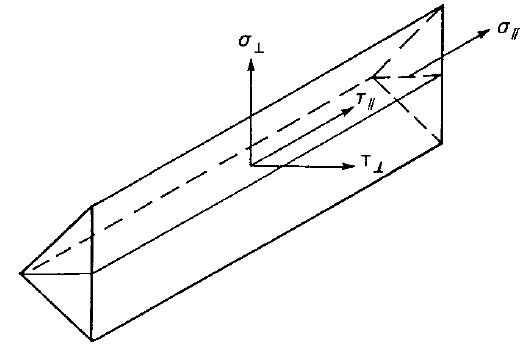CJP groove weldsThe resistance of Complete Joint Penetration (CJP) groove welds is assumed as that of the base metal. Check of bolts and preloaded bolts according to CISC The forces in bolts including prying forces are determined by finite element analysis. The bolt resistances are checked by S16 – Chapter 13.BoltsTensile strength of boltsThe tensile resistance of a bolt is assessed according to Clause 13.12.1.3 and taken as:$T_r = 0.75 \phi_b A_b F_u$where: ϕb = 0.8 – resistance factor for bolts, editable in Code setup Ab – cross-sectional area of a bolt based on its nominal diameter Fu – specified minimum tensile strength for a bolt When the bolt threads are intercepted by a shear plane, the shear resistance is taken as 0.7 Vr.Shear strength of boltsThe shear resistance of a bolt is assessed according to Clause 13.12.1.2. Each shear plane of a bolt is checked separately. It is taken as:$V_r=0.6 \phi_b A_b F_u$where: ϕb = 0.8 – the resistance factor for bolts, editable in Code setup Ab – cross-sectional area of a bolt based on its nominal diameter Fu – specified minimum tensile strength for a bolt When the bolt threads are intercepted by a shear plane, the shear resistance is taken as 0.7 Vr.Combined tension and shear in bearing type connectionThe resistance of a bolt loaded by combined tension and shear is assessed according to Clause 13.12.1.4 and taken as:$\left ( \frac{V_f}{V_r} \right )^2 + \left ( \frac{T_f}{T_r} \right )^2 \le 1$where: Vf and Tf are design shear force and tensile force acting on the bolt, respectively Vr and Tr are design shear resistance and tensile resistance of the bolt, respectively Bearing strength in bolt holesThe resistance developed at the bolt in a bolted joint subjected to bearing and shear is assessed according to Clause 13.12.1.2 and taken asBr = 3 ϕbr t d Fu for regular bolt holesBr = 2.4 ϕbr t d Fu for slotted holes loaded perpendicular to these holeswhere: ϕbr = 0.8 – resistance factor for bearing of bolts on steel t – thinner thickness of connected plates d – diameter of a bolt Fu – tensile strength of the connected material Hole tear-out of a boltThe resistance of hole tear-out of a bolt is checked for individual bolts according to Clause 13.11 as:$T_r = \phi_u 0.6 A_{gv} \frac{F_y+F_u}{2}$where: ϕu = 0.75 – resistance factor for structural steel Agv = 2 ∙ l ∙ t – gross area in shear Fy – yield strength of the connected material Fu – tensile strength of the connected material l – distance from centreline of the bolt to the edge in the direction of the shear force t – thickness of the connected material For steel grades with Fy > 460 MPa, (Fy + Fu) / 2 shall be replaced with Fy in the determination of Tr.Bolts in slip-critical connectionsThe slip resistance of a bolted joint is assessed according to Clause 13.12.2 asVs = 0.53 cs ks Ab Fuwhere: cs – coefficient determined according to ks and bolt grade: for ks < 0.52 class A cs = 1.00 (A325) or 0.92 (A490) or 0.78 (other) for ks ≥ 0.52 class B cs = 1.04 (A325) or 0.96 (A490) or 0.81 (other) ks – friction coef. editable in Code setup which should be set according to Table 3 in S16-14; equals 0.3 for class A or 0.52 for class B Ab – cross-sectional area of a bolt based on its nominal diameter Fu – specified minimum tensile strength for a bolt When slotted holes are used in slip-critical connections, Vs = 0.75 ∙ 0.53 cs ks Ab Fu.A bolt subjected to both tension and shear must satisfy the following relationship:$\frac{V_f}{V_s}+1.9\frac{T}{A_b F_u}$where: Vf and Tf are the design shear force and the tensile force acting on the bolt, respectively Clause 13.12.2 states that the resistances of the connection as specified in Clause 13.12.1 shall be checked. The user should, therefore, check the state after slip occurs, i.e. change the shear force transfer of bolts from “Friction” to “Bearing – tension and shear interaction”.DetailingIn the detailing of bolted connections, the minimum pitch and minimum edge distance are checked according to S16-14 – 22.3. Minimum pitch (2.7 d – editable in Code setup) and minimum edge distance (1.25 d) are checked. Check of concrete block according to CISC Concrete below base plate is simulated by Winkler subsoil with uniform stiffness, which provides the contact stresses. The average stress at the loaded area in contact with base plate is used for compressive check.Concrete in compressionThe concrete design bearing strength in compression is determined in accordance with S16-14 – 25.3.1 and CSA A23.3 – 10.8. When the supported surface of the concrete is larger than the base plate the design bearing strength is defined as$f_{p,(max)} = 0.85 \phi_c f'_c \sqrt{\frac{A_2}{A_1}} \le 1.7 \phi_c f'_c$where: ϕc=0.65 – resistance factor for concrete f'c – concrete compressive strength A1 – base plate area in contact with concrete surface (upper surface area of the frustum) A2 – concrete supporting surface (geometrically similar lower area of the frustum having its slopes of 1 vertical to 2 horizontal) The assessment of concrete in bearing is as follows:σ ≤ fp(max)where: σ – average compressive stress under the base plate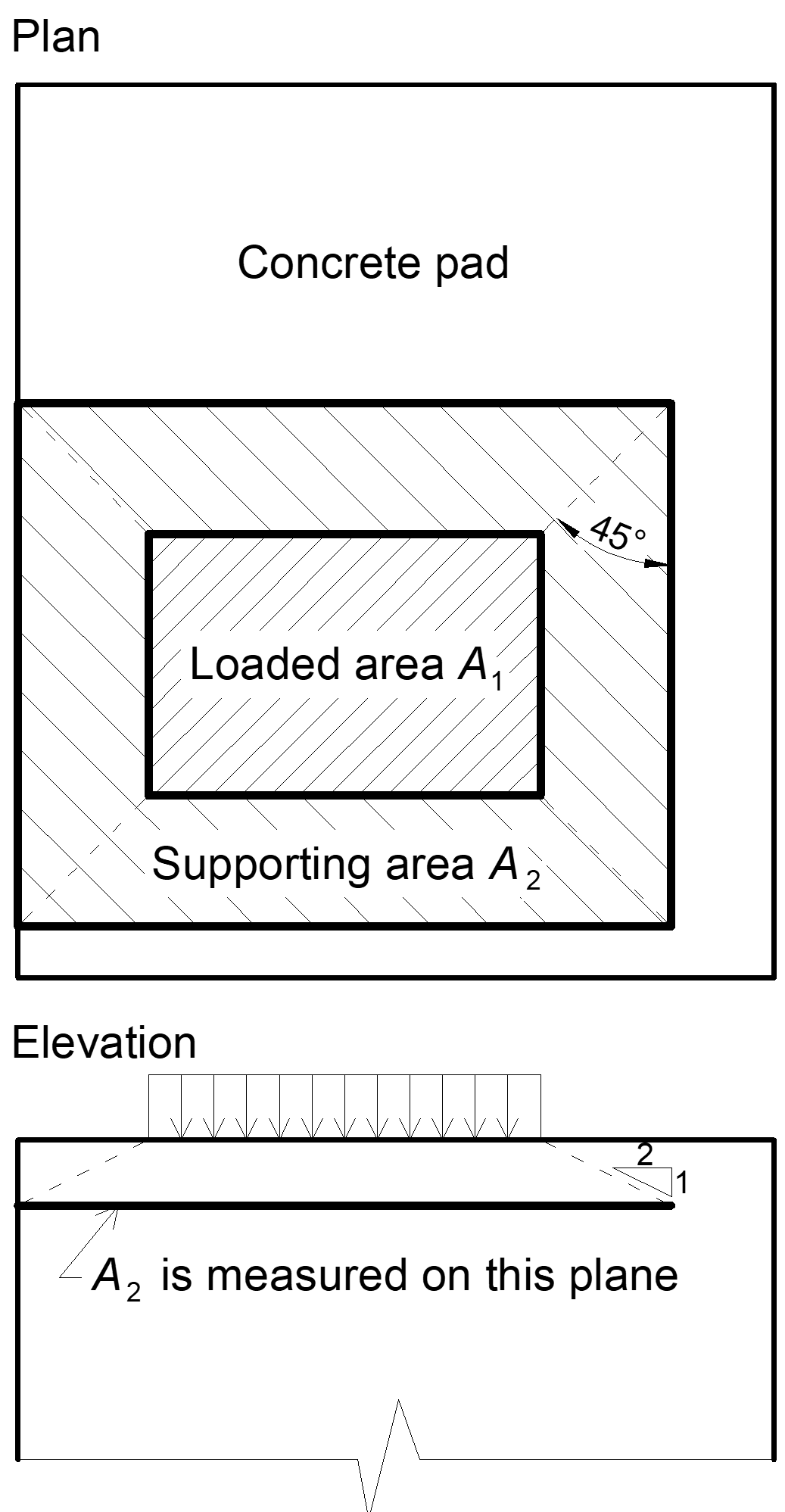Transfer of shear forcesShear loads can be transferred via one of these options: Shear lug, Friction, Anchor bolts. Shear lugShear loads are considered to be transferred only via shear lug. Concrete bearing is not checked in software and should be checked by user elsewhere. Shear lug and welds are checked using FEM and weld components.FrictionIn case of compressive force, the shear loads can be transferred via friction between a concrete pad and a base plate. The friction coefficient is editable in Code setup.Anchor boltsIf the shear load is transferred via anchor bolts only, the shear force acting on each anchor is determined by FEA and anchor bolts are assessed according to ACI 318-14 as described in following chapters. Check of anchors according to CISC The forces in anchors including prying forces are determined by finite element analysis, but the resistances are checked using code provisions of A23.3 - Annex D.Anchor rods are designed according to A23.3-14 – Annex D. The following resistances of anchor bolts are evaluated: Steel strength of anchor in tension Nsar, Concrete breakout strength in tension Ncbr, Concrete pullout strength Npr, Concrete side-face blowout strength Nsbr, Steel strength of anchor in shear Vsar, Concrete breakout strength in shear Vcbr, Concrete pryout strength of anchor in shear Vcpr. The concrete condition may be chosen by user as cracked or non-cracked. The type of anchors (cast-in headed with circular or rectangular washers, straight anchors) is selected by user, the pullout strength and side-face blowout strength is checked in the software only for headed anchors.Steel resistance of anchor in tensionSteel strength of anchor in tension is determined according to CSA A23.3-14 – D.6.1 asNsar = Ase,N ϕs futa Rwhere: ϕs = 0.85 – steel embedment material resistance factor for reinforcement Ase,N – effective cross-sectional area of an anchor in tension futa ≤ min (860 MPa, 1.9 fya) – specified tensile strength of anchor steel fya – specified yield strength of anchor steel R = 0.8 – resistance modification factor as specified in CSA A23.3.-14 – D.5.3 Concrete breakout resistance of anchor in tensionConcrete breakout strength is designed according to the Concrete Capacity Design (CCD) in CSA A23.3-14 – D.6.2. In the CCD method, the concrete cone is considered to be formed at an angle of approximately 34° (1 vertical to 1.5 horizontal slope). For simplification, the cone is considered to be square rather than round in plan. The concrete breakout stress in the CCD method is considered to decrease with an increase in size of the breakout surface.$N_{cbrg} = \frac{A_{Nc}}{A_{Nco}} \psi_{ed,N} \psi_{ec,N} \psi_{c,N} N_{br}$where: ANc – concrete breakout cone area for group of anchors loaded by tension that create common concrete cone ANco = 9 hef2 – concrete breakout cone area for single anchor not influenced by concrete edges $$\psi_{ed,N} = \min \left ( 0.7+\frac{0.3 c_{a,min}}{1.5 h_{ef}}, \, 1 \right )$$– modification factor for edge distance ca,min – the smallest distance from the anchor to the edge hef – depth of embedment; according to A23.3-14 – D.6.2.3, the effective embedment depth hef is reduced to $$h_{ef} = \max \left ( \frac{c_{a,max}}{1.5}, \, \frac{s}{3} \right )$$ if anchors are located less than 1.5 hef from three or more edges $$\psi_{ec,N} = \frac{1}{1+\frac{2e'_N}{3 h_{ef}}}$$ – modification factor for eccentrically loaded group of anchors e'N – tension load eccentricity with respect to the center of gravity of anchors loaded by tension and creating a common concrete cone Ψc,N – modification factor for concrete conditions; Ψc,N = 1 for cracked concrete, Ψc,N = 1.25 for non-cracked concrete $$N_{br} = k_c \phi_c \lambda_a \sqrt{f'_c} h_{ef}^{1.5} R$$ – basic concrete breakout strength of a single anchor in tension in cracked concrete; for cast-in headed anchors and 275 mm ≤ hef ≤ 625 mm, $$N_{br} = 3.9 \phi_c \lambda_a \sqrt{f'_c} h_{ef}^{5/3} R$$ ϕc=0.65 – resistance factor for concrete kc=10 for cast-in anchors s – spacing between anchors ca,max – maximum distance from an anchor to one of the three close edges λa = 1 – is modification factor for lightweight concrete f'c – concrete compressive strength [MPa] R = 1 – resistance modification factor as specified in CSA A23.3 – D.5.3 According to A23.3-14 – D.6.2.8, in case of headed anchors, the projected surface area ANc is determined from the effective perimeter of the washer plate, which is the lesser value of da + 2 twp or dwp, where: da – anchor diameter dwp – washer plate diameter or edge size twp – washer plate thickness The group of anchors is checked against the sum of tensile forces in anchors loaded in tension and creating a common concrete cone.The concrete breakout cone area for group of anchors loaded by tension that create common concrete cone, Ac,N, is shown by red dashed line.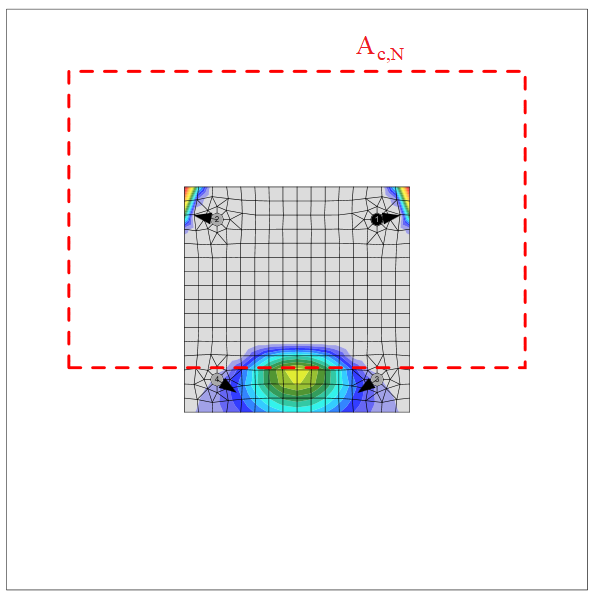According to CSA A23.3-14 – D.6.2.9, where anchor reinforcement is developed in accordance with Clause 12 of A23.3-14 on both sides of the breakout surface, the anchor reinforcement is presumed to transfer the tension forces and concrete breakout strength is not evaluated (can be set in Code setup).Concrete pullout resistance of anchor in tensionConcrete pullout strength of a headed anchor is defined in CSA A23.3-14 – D.6.3 asNcpr = Ψc,P Nprwhere: Ψc,P – modification factor for concrete condition; Ψc,P = 1.0 for cracked concrete, Ψc,P = 1.4 for non-cracked concrete Npr = 8 Abrg ϕc f'c R for headed anchor Abrg – bearing area of the head of stud or anchor bolt ϕc = 0.65 – resistance factor for concrete da – anchor diameter f'c – concrete compressive strength R = 1 – resistance modification factor as specified in CSA A23.3 – D.5.3 Concrete pullout strength for other types of anchors than headed is not evaluated in the software and has to be specified by the manufacturer.Concrete side-face blowout resistanceConcrete side-face blowout strength of headed anchor in tension is defined in CSA A23.3-14 – D.6.4 as:$N_{sbr} = 13.3 c_{a1} \sqrt{A_{brg}} \phi_c \lambda_a \sqrt{f'_c} R$If ca2 for the single anchor loaded in tension is less than 3 ca1, the value of Nsbr is multiplied by the factor 0.5 ≤ (1+ ca2 / ca1) / 4 ≤ 1.A group of headed anchors with deep embedment close to an edge (hef > 2.5 ca1) and spacing between anchors less than 6 ca1 has the strength:$N_{sbgr} = \left (1 + \frac{s} {6 c_{a1}} \right ) N_{sbr}$Only one reduction factor at a time is applied.where: ca1 – the shorter distance from an anchor to an edge ca2 – the longer distance, perpendicular to ca1, from an anchor to an edge Abrg – a bearing area of the head of stud or anchor bolt ϕc – resistance factor for concrete editable in Code setup f'c – concrete compressive strength hef – depth of embedment; according to A23.3-14 – D.6.2.3, the effective embedment depth hef is reduced to $$h_{ef} = \max \left ( \frac{c_{a,max}}{1.5}, \, \frac{s}{3} \right )$$ if anchors are located less than 1.5 hef from three or more edges s – spacing between anchors R = 1 – resistance modification factor as specified in CSA A23.3 – D.5.3 Steel resistance of anchor in shearThe steel strength in shear is determined according to A23.3 – D.7.1 asVsar = Ase,V ϕs 0.6 futa Rwhere: ϕs = 0.85 – steel embedment material resistance factor for reinforcement Ase,V – effective cross-sectional area of an anchor in shear futa – specified tensile strength of anchor steel but not greater than the smaller of 1.9 fya or 860 MPa R = 0.75 – resistance modification factor as specified in CSA A23.3 – D.5.3 If mortar joint is selected, steel strength in shear Vsa is multiplied by 0.8 (A23.3 –D.7.1.3).The shear on lever arm, which is present in case of base plate with oversized holes and washers or plates added to the top of the base plate to transmit the shear force, is not considered.Concrete breakout resistance of anchor in shearConcrete breakout strength of an anchor in shear is designed according to A23.3 –D.7.2. The shear force acting on a base plate is assumed to be transferred by the anchors which are closest to the edge in the direction of the shear force. The direction of the shear force with respect to the concrete edge affects the concrete breakout strength according to FIB Bulletin 58 – Design of anchorages in concrete – Guide to good practice (2011). If concrete cones of anchors overlap, they create a common concrete cone. The eccentricity in shear is also taken into account.$V_{cbr} = \frac{A_{Vc}}{A_{Vco}} \psi_{ec,V} \psi_{ed,V} \psi_{c,V} \psi_{h,V} \psi_{\alpha,V} V_{br}$where: AVc – projected concrete failure area of an anchor or group of anchors divided by number of anchors in this group AVco = 4.5 ca12 – projected concrete failure area of one anchor when not limited by corner influences, spacing or member thickness $$\psi_{ec,V} = \frac{1}{1+ \frac{2 e'_V}{3c_{a1}}}$$ – modification factor for group of anchors loaded eccentrically in shear $$\psi_{ed,V} = 0.7 + 0.3 \frac{c_{a2}}{1.5 c_{a1}}\le1.0$$– modification factor for edge effect Ψc,V – modification factor for concrete condition; Ψc,V = 1.0 for cracked concrete, Ψc,V = 1.4 for non-cracked concrete $$\psi_{h,V}=\sqrt{\frac{1.5c_{a1}}{h_a}} \ge 1$$– modification factor for anchors located in a concrete member where ha < 1.5 ca1 $$\psi_{\alpha,V} = \sqrt{\frac{1}{(\cos \alpha_V)^2+(0.5\sin \alpha_V)^2}}$$ – modification factor for anchors loaded at an angle with the concrete edge (FIB Bulletin 58 – Design of anchorages in concrete – Guide to good practice, 2011) ha – height of a failure surface on the concrete side $$V_{br}=\min⁡ \left(0.58 \left (\frac{l_e}{d_a} \right )^{0.2} \sqrt{d_a} \phi_c \lambda_a \sqrt{f'_c} c_{a1}^{1.5} R, \, 3.75 \lambda_a \phi_c \sqrt{f'_c} c_{a1}^{1.5} R \right )$$ le = hef ≤ 8 da – load-bearing length of the anchor in shear da – anchor diameter f'c – concrete compressive strength ca1 – edge distance in the direction of load; according to Cl. 17.5.2.4, for a narrow member, c2,max < 1.5 c1 that is also deemed to be thin, ha < 1.5 c1, c'1 is used in previous equations instead of c1; the reduced c'1 = max (c2,max / 1.5, ha / 1.5, sc,max / 3) ca2 – edge distance in the direction perpendicular to load c2,max – largest edge distance in the direction perpendicular to load sc,max – maximum spacing perpendicular to direction of shear, between anchors within a group ϕc = 0.65 – resistance factor for concrete R = 1 – resistance modification factor as specified in CSA A23.3 – D.5.3 If both edge distances ca2 ≤ 1.5ca1 and ha ≤ 1.5 ca1, $$c_{a1} = \max \left ( \frac{c_{a2}}{1.5}, \, \frac{h_a}{1.5}, \, \frac{s}{3} \right )$$, where s is the maximum spacing perpendicular to direction of shear, between anchors within a group.According to A23.3-14 – D.7.2.9, where anchor reinforcement is developed in accordance with A23.3-14 – Clause 12 on both sides of the breakout surface, the anchor reinforcement is presumed to transfer the shear forces and concrete breakout strength is not evaluated.Concrete pryout resistance of an anchor in shearConcrete pryout strength is designed according to A23.3 – D.7.3.Vcpr = kcp Ncprwhere: kcp = 1.0 for hef < 65 mm, kcp = 2.0 for hef ≥ 65 mm Ncpr – concrete breakout strength – all anchors are considered to be in tension According to CSA A23.3-14 – D.6.2.9, where anchor reinforcement is developed in accordance with Clause 12 of A23.3-14 on both sides of the breakout surface, the anchor reinforcement is presumed to transfer the tension forces and concrete breakout strength is not evaluated (can be set in Code setup).Interaction of tensile and shear forcesInteraction of tensile and shear forces is assessed according to A23.3 – Figure D.18.$\left ( \frac{N_f}{N_r} \right )^{5/3}+\left ( \frac{V_f}{V_r} \right )^{5/3} \le 1.0$where: Nf and Vf – design forces acting on an anchor Nr and Vr – the lowest design strengths determined from all appropriate failure modes Anchors with stand-offAnchor with stand-off is designed as a bar element loaded by shear force, bending moment and compressive or tensile force. These internal forces are determined by finite element model. The anchor is fixed on both sides, one side is 0.5×d below the concrete level, the other side is in the middle of the thickness of the plate. The buckling length is conservatively assumed as twice the length of the bar element. Plastic section modulus is used. The bar element is designed according to S16-14. Interaction of shear force is neglected because the minimum length of the anchor to fit the nut under the base plate ensures that the anchor fails in bending before the shear force reaches half the shear resistance and the shear interaction is negligible (up to 7 %). Interaction of bending moment and compressive or tensile force is conservatively assumed as linear. Second order effects are not taken into account.Shear resistance (CSA S16-14 – 13.4.4):Vr = ϕ ∙ 0.66 ∙ Av ∙ Fy Av = 0.844 ∙ As – the shear area As – the bolt area reduced by threads Fy – bolt yield strength ϕ – the resistance factor, recommended value is 0.9 Tensile resistance (CSA S16-14 – 13.2)Tr = ϕ ∙ As ∙ FyCompressive resistance (CSA S16-14 – 13.3.1)$C_r = \frac{\phi A_s F_y}{\left (1+\lambda^{2n}\right )^{\frac{1}{n}}}$ $$\lambda = \sqrt{\frac{F_y}{F_e}}$$ – anchor bolt slenderness $$F_e = \frac{\pi^2 E}{\left (\frac{KL}{r}\right )^2}$$ – elastic buckling stress KL = 2 ∙ l – buckling length l – length of the bolt element equal to half the base plate thickness + gap + half the bolt diameter $$r = \sqrt{\frac{I}{A_s}}$$ – radius of gyration of the anchor bolt $$I=\frac{\pi d_s^4}{64}$$– moment of inertia of the bolt n = 1.34 – parameter for compressive resistance Bending resistance (CSA S16-14 – 13.5):Mr = ϕ ∙ Z ∙ FyZ = ds3 / 6 – plastic section modulus of the boltLinear interaction:$$\frac{N}{C_r}+\frac{M}{M_r} \le 1$$ ... for compressive normal force$$\frac{N}{T_r}+\frac{M}{M_r} \le 1$$ ... for tensile normal force N – tensile (positive) or compressive (negative sign) factored force Cr – factored compressive (negative sign) resistance Tr – factored tensile (positive sign) resistance M – factored bending moment Mr – factored moment resistance DetailingThe spacing between anchors should be greater than 4 times anchor diameter according to A23.3-14 – D.9.2. Joint classification according to CISC Joints are classified according to joint stiffness to: Rigid – joints with insignificant change of original angles between members, Semirigid – joints which are assumed to have the capacity to furnish a dependable and known degree of flexural restraint, Simple – joints which do not develop bending moments. Canadian standard S14-16, Cl. 8.2 does not provide exact boundaries so the joints are classified according to the commentary in AISC 360-16, Cl. B3.4. Rigid – $$\frac{S_{j,ini} L_b}{E I_b} \ge 20$$ Semirigid – $$2 < \frac{S_{j,ini} L_b}{E I_b} < 20$$ Simple – $$\frac{S_{j,ini} L_b}{E I_b} \le 2$$ where: Sj,ini – initial stiffness of the joint; the joint stiffness is assumed linear up to the 2/3 of Mj,Rd Lb – theoretical length of the analyzed member E – Young's modulus of elasticity Ib – moment of inertia of the analyzed member Mj,Rd – joint design moment resistance Capacity design according to CISC Capacity design is a part of seismic check and ensures that the joint has sufficient deformation capacity.The objective of capacity design is to confirm a building undergoes controlled ductile behaviour in order to avoid collapse in a design-level earthquake. Plastic hinge is expected to appear in dissipative item and all non-dissipative items of the joint must be able to safely transfer forces due to the yielding in the dissipative item. The dissipative item is usually a beam in moment resisting frame but it may also be e.g. an end plate. The resistance factor is not used for dissipative items. Two factors are assigned to the dissipative item: Ry = 1.1 – overstrength factor – S16-14, Cl. 27.1.7; editable in materials Cpr = 1.1 – strain-hardening factor – S16-14, Cl. 27.2.2; it is recommended to apply for beam as a dissipative item in moment resisting frame The material diagram is modified according to the following figure: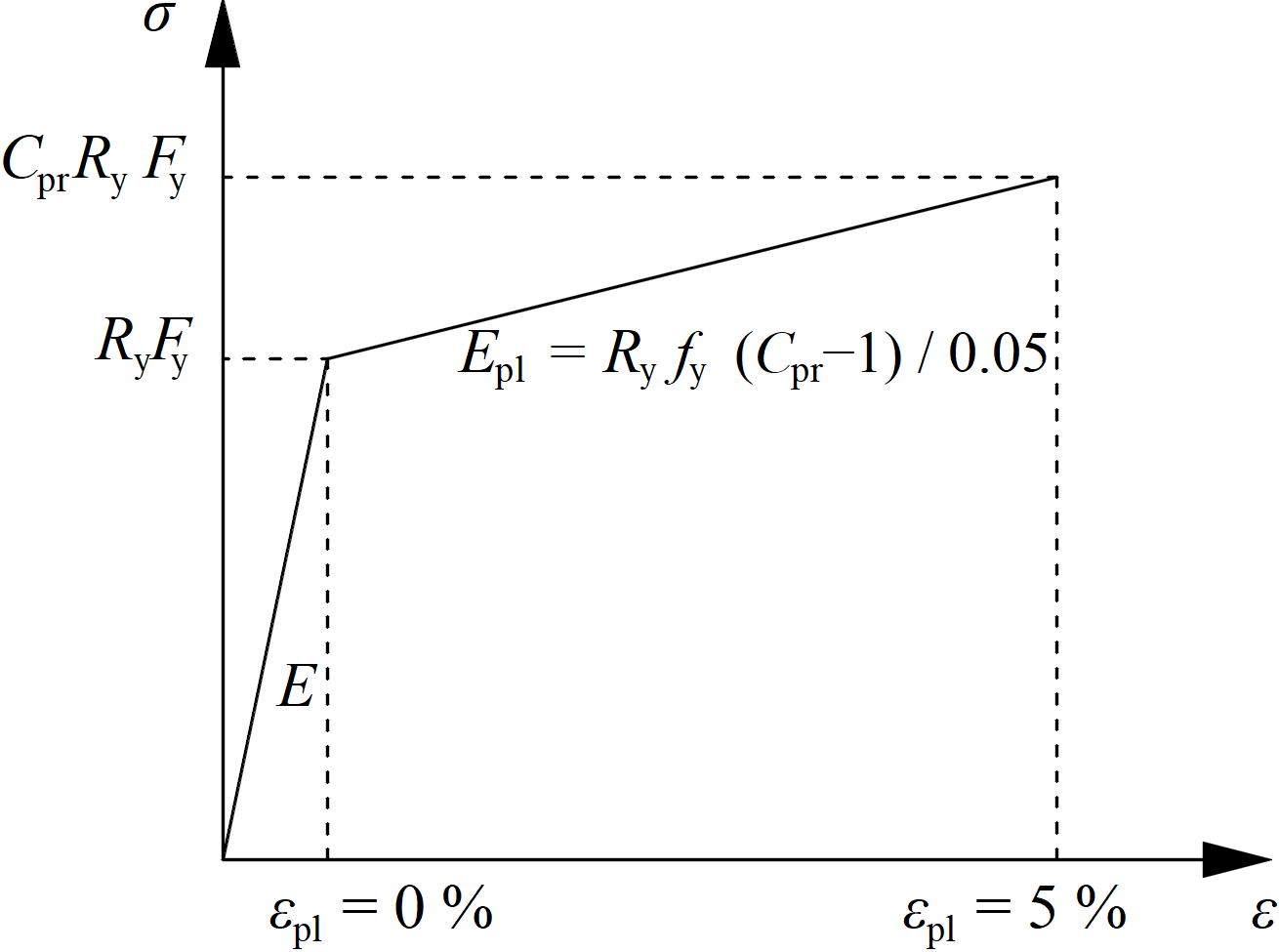The increased strength of the dissipative item allows for the input of loads that cause the plastic hinge to appear in the dissipative item. In the case of moment resisting frame and beam as the dissipative item, the beam should be loaded by My = CprRyFyWpl,y and corresponding shear forceVz = –2 My,Ed / Lh, where: Fy – yield strength Wpl,y – plastic section modulus Lh – distance between plastic hinges on the beam In case of asymmetric joint, the beam should be loaded by both sagging and hogging bending moments and their corresponding shear forces.The plates of dissipative items are excluded from check.$$